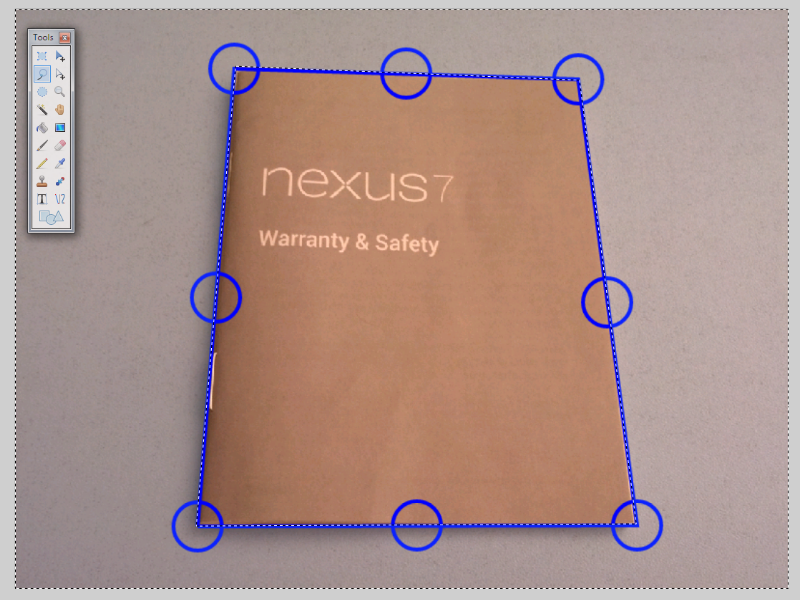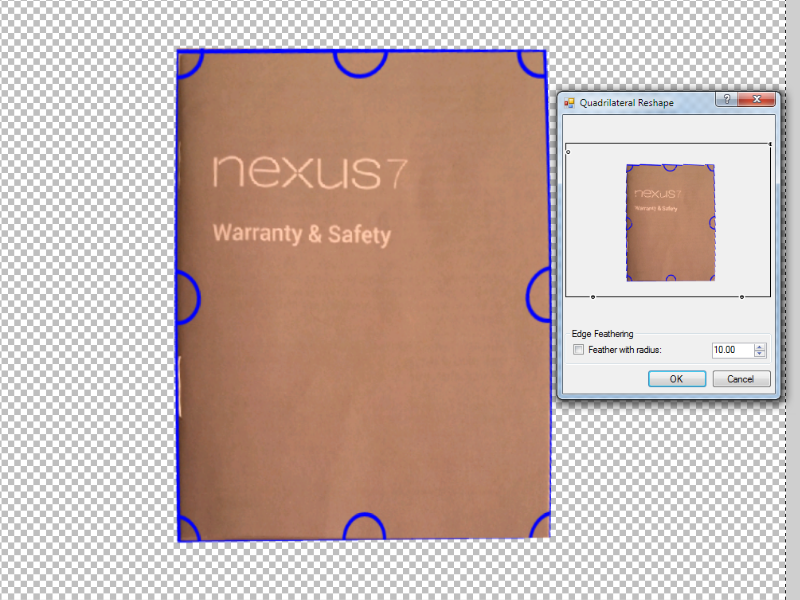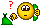# How to crop to document edges in photo?

## Recommended Posts

Hi there,

I was wondering if there was a way in paint.net to crop a photo of a document to the documents edges, like the many mobile apps for example:

This is actually a pretty hard thing to search for, no idea of what terms to search for.Edited by ttOM123
##### Share on other sites

You can use the Quadrilateral Reshape plugin to adjust the perspective. Then, just select with rectangular marquee and hit ctrl+shift+x to crop to the selection.

No, Paint.NET is not spyware...but, installing it is an IQ test. ~BoltBait

Blend modes are like the filling in your sandwich. It's the filling that can change your experience of the sandwich. ~Ego Eram Reputo

##### Share on other sites

Well there is no easy way to do the job. I usually use the select tool and then press delete. Unfortunaly, you will be left with a skewed shape.I wish there was an easy way to correct the perspective distortion and have square corners but I have not seen a tool to do it. Nevertheless you can try and play with the "Perspective effect" to square it.

Edit: After playing with pdnoob's advise you can get something like this :Edited by Eli
##### Share on other sites

You can use the Quadrilateral Reshape plugin to adjust the perspective. Then, just select with rectangular marquee and hit ctrl+shift+x to crop to the selection.

Tried Quadrilateral Reshape to make rectangular then a rectangular crop, works ok I guess but is quite fiddly. I was hoping for a "Quadrilateral" select tool, which then from edges makes rectangular and crops.

##### Share on other sites

Well there is no easy way to do the job. I usually use the select tool and then press delete. Unfortunaly, you will be left with a skewed shape.

....

Yeah I got an similar effect by using Quadrilateral Reshape to get rectangular then cropping. I've been looking for the last few hours on how to do this nicely in Windows in any program but haven't found that much.

I have never looked into image processing but if one could list the coordinates of a quadrilateral shape, then apply some translation of the pixels to some normalized rectangle, it might work. Probably not that simple I guess.

##### Share on other sites

... I have never looked into image processing but if one could list the coordinates of a quadrilateral shape, then apply some translation of the pixels to some normalized rectangle, it might work. Probably not that simple I guess.

Something like Quadrilateral crop (I haven't thought out all cases):

2) Find the 2 diagonal points of Quadrilateral with the smallest distance. Call them p1 = (x1, y1), p2 = (x2, y2). The other point closest horizontally to p1 is called p3 = (x3, y3), remaining point is p4 = (x4, y4)

3) these points p1 p2 remain the same after some translation. These new points once translated become P1 = (x1, y1), P2 = (x2, y2)

4) The other diagonal pair p3 = (x3, y3), p4 = (x4, y4) get mapped to P3 = (x1, y2), P4 = (x2, y1).

5) Apply crop to rectangle P1 P2 P3 P4Edited by ttOM123
##### Share on other sitesPlease hold. I will transfer you to the math department.•1
##### Share on other sites

Well there is no easy way to do the job. I usually use the select tool and then press delete. Unfortunaly, you will be left with a skewed shape.I wish there was an easy way to correct the perspective distortion and have square corners but I have not seen a tool to do it. Nevertheless you can try and play with the "Perspective effect" to square it.

I don't know much about plug-ins but I can imagine why it might do that if it's based on pixel positions. Say for example, the upper left pixel position ends with a 4, and the bottom left one 9, the middle pixel would be 6.5.... except I don't think half a pixel is possible lol. It probably has to go to the next lowest full pixel if its on the left and the next highest full pixel if on the right. If set to just the 4 corner positions without the middle, it could work without skewing it, that is if it works the way I think it might.

Edited by RFX##### Share on other sites

For this situation, fractional pixels are possible. A plugin that performed the inverse perspective transformation needed would probably use a function (or method, if you prefer) called "GetBilinearSample" which allows fractional pixel values. The color is interpolated between the actual pixels in the source buffer. This results in some degree of blurring, but for most images it would be very acceptable.

##### Share on other sites

I'm working on some other things at the time, but when I get a chance (assuming no one beats me to it), I'll try to write a plugin to help with this problem.

•1
##### Share on other sites

Yeah time, the most limiting resource. I would also look into it if I wasn't also busy.

Is it possible to feed the Quadrilateral Reshape plugin a set of points, or do you need the source code? It seems to do the transform and interpolation already.

##### Share on other sites

Is it possible to feed the Quadrilateral Reshape plugin a set of points, or do you need the source code? It seems to do the transform and interpolation already.

Are you saying you would want to manually type in values for the 4 points? If so, I think that would be possible. Otherwise, please elaborate.My Gallery  |  My Plugin Pack

##### Share on other sites

The perspective matrix that maps {(0,0), (1,0), (1,1), (0,1)} to {(x0,y0), (x1,y1), (x2,y2), (x3,y3)} is:

```  / x' \     / T230 * x1 - T123 * x0     T012 * x3 - T123 * x0      T123 * x0 \   / x \
|  y'  | = |  T230 * y1 - T123 * y0     T012 * y3 - T123 * y0      T123 * y0  | |  y  |
\ w' /     \      T230 - T123               T012 - T123             T123    /   \ 1 /

| x2-x3  x0-x3 |           | x1-x2  x3-x2 |           | x0-x1   x2-x1 |
Where T230 = |              |    T123 = |              |    T012 = |               |
| y2-y3  y0-y3 |           | y1-y2  y3-y2 |           | y0-y1   y2-y1 |

(x", y") = (x'/w', y'/w')

I.e,

x' = (T230 * x1 - T123 * x0) * x + (T012 * x3 - T123 * x0) * y + T123 * x0
y' = (T230 * y1 - T123 * y0) * x + (T012 * y3 - T123 * y0) * y + T123 * y0
w' = (T230 - T123) * x + (T012 - T123) * y + T123

```
(The determinants can be written quite a few different ways.)

These are what are called homogeneous coordinates. The coordinates to be transformed are assumed to have a 1 as the third component. Once the coordinates are transformed, the x and y values are divided by the third component to complete the transformation.

Edited by MJW
##### Share on other sites

Are you saying you would want to manually type in values for the 4 points? If so, I think that would be possible. Otherwise, please elaborate.

Yes, I was thinking noting the 4 edges of the Quadrilateral then enter them somehow.

##### Share on other sites

The perspective matrix that maps {(0,0), (1,0), (1,1), (0,1)} to {(x0,y0), (x1,y1), (x2,y2), (x3,y3)} is:

```  / x' \     / T230 * x1 - T123 * x0     T012 * x3 - T123 * x0      T123 * x0 \   / x \
|  y'  | = |  T230 * y1 - T123 * y0     T012 * y3 - T123 * y0      T123 * y0  | |  y  |
\ w' /     \      T230 - T123               T012 - T123             T123    /   \ 1 /

```

I'm curious as how you got this transformation matrix. Do you have any diagrams or something?

Looks like OpenCV uses this method:

This explantion here doesn't look too bad: http://stackoverflow.com/a/3190725/2750236

so my take on it: we know for n=4 (x1,y1), (x2,y2), (x3,y3), (x4,y4) which are our rectangle edges we are transforming to and (X1, Y1) ... (X4, Y4) which are the defined Quadrilateral edges.Solve for the vector a ... h

then transforming from a rectangle to a Quadrilateral isin fact this method looks similar to your method haha. With this method, I still can't see how they get the transformation matrix.

Edited by ttOM123
##### Share on other sites

Installed Codelab, made a rough script but nothing changes? Not sure why? Can you print out values to check where the pixels get mapped to? I'm trying to fix the photo to the booklet in the first post. EDIT: yes looks like its mapping to out of bounds regions```// Name:
// Author:
// Title:
// Desc:
// Keywords:
// URL:
// Help:
#region UICode
int Amount1=0;	//[0,100]Slider 1 Description
int Amount2=0;	//[0,100]Slider 2 Description
int Amount3=0;	//[0,100]Slider 3 Description
#endregion

void Render(Surface dst, Surface src, Rectangle rect)
{
// Delete any of these lines you don't need
Rectangle selection = EnvironmentParameters.GetSelection(src.Bounds).GetBoundsInt();
int CenterX = ((selection.Right - selection.Left) / 2)+selection.Left;
int CenterY = ((selection.Bottom - selection.Top) / 2)+selection.Top;
ColorBgra PrimaryColor = (ColorBgra)EnvironmentParameters.PrimaryColor;
ColorBgra SecondaryColor = (ColorBgra)EnvironmentParameters.SecondaryColor;
int BrushWidth = (int)EnvironmentParameters.BrushWidth;

ColorBgra CurrentPixel;

double  a =  3.7;
double  b = 0.3;
double  c = -1912.5;
double  d = 0;
double  e = -1.8;
double  f = 1149.9;
double  g = 0;
double  h = 0;
double  X, Y;
int XX, YY;

for (int y = rect.Top; y < rect.Bottom; y++)
{
if (IsCancelRequested) return;
for (int x = rect.Left; x < rect.Right; x++)
{
CurrentPixel = src[x,y];
X = (a*x + b*y + c) / (g*x + h*y + 1);
Y = (d*x + e*y + f) / (g*x + h*y + 1);
XX =  Convert.ToInt32(X);
YY =  Convert.ToInt32(Y);
if (XX  < rect.Right) {
if (XX  > rect.Left) {
if (YY < rect.Top) {
if (YY > rect.Bottom) {
dst[XX,YY] = CurrentPixel;
}
}
}
}
}
}
}

```
Edited by ttOM123
##### Share on other sites

My math was wrong; these numbers for a to h should now work, but still script isn't doing anything:

```// Name:
// Author:
// Title:
// Desc:
// Keywords:
// URL:
// Help:
#region UICode
int Amount1=0;	//[0,100]Slider 1 Description
int Amount2=0;	//[0,100]Slider 2 Description
int Amount3=0;	//[0,100]Slider 3 Description
#endregion

void Render(Surface dst, Surface src, Rectangle rect)
{
// Delete any of these lines you don't need
Rectangle selection = EnvironmentParameters.GetSelection(src.Bounds).GetBoundsInt();
int CenterX = ((selection.Right - selection.Left) / 2)+selection.Left;
int CenterY = ((selection.Bottom - selection.Top) / 2)+selection.Top;
ColorBgra PrimaryColor = (ColorBgra)EnvironmentParameters.PrimaryColor;
ColorBgra SecondaryColor = (ColorBgra)EnvironmentParameters.SecondaryColor;
int BrushWidth = (int)EnvironmentParameters.BrushWidth;

ColorBgra CurrentPixel;
double a = 0.375007;
double b = 0.214097;
double c = 471.000000;
double d = 0.014862;
double e = -0.531855;
double f = 640.000000;
double g = 0.000022;
double h = 0.000337;
double  X, Y;
int XX, YY;

for (int y = rect.Top; y < rect.Bottom; y++)
{
if (IsCancelRequested) return;
for (int x = rect.Left; x < rect.Right; x++)
{
CurrentPixel = src[x,y];
X = (a*x + b*y + c) / (g*x + h*y + 1);
Y = (d*x + e*y + f) / (g*x + h*y + 1);
XX =  Convert.ToInt32(X);
YY =  Convert.ToInt32(Y);
if (XX  < rect.Right) {
if (XX  > rect.Left) {
if (YY < rect.Top) {
if (YY > rect.Bottom) {
dst[XX,YY] = CurrentPixel;
}
}
}
}
}
}
}
```
##### Share on other sites

YES got it working!! Sorry for all the spamming posts. All my if checks are not working for some reason, not sure why?

Here's the first result, I copied the photo into paint.net and run the script:```// Name:
// Author:
// Title:
// Desc:
// Keywords:
// URL:
// Help:
#region UICode
int Amount1=0;	//[0,100]Slider 1 Description
int Amount2=0;	//[0,100]Slider 2 Description
int Amount3=0;	//[0,100]Slider 3 Description
#endregion

void Render(Surface dst, Surface src, Rectangle rect)
{
// Delete any of these lines you don't need
Rectangle selection = EnvironmentParameters.GetSelection(src.Bounds).GetBoundsInt();
int CenterX = ((selection.Right - selection.Left) / 2)+selection.Left;
int CenterY = ((selection.Bottom - selection.Top) / 2)+selection.Top;
ColorBgra PrimaryColor = (ColorBgra)EnvironmentParameters.PrimaryColor;
ColorBgra SecondaryColor = (ColorBgra)EnvironmentParameters.SecondaryColor;
int BrushWidth = (int)EnvironmentParameters.BrushWidth;

ColorBgra CurrentPixel;
double a = 0.375007;
double b = 0.214097;
double c = 471.000000;
double d = 0.014862;
double e = -0.531855;
double f = 640.000000;
double g = 0.000022;
double h = 0.000337;
double  X, Y;
int XX, YY;

for (int y = rect.Top; y < rect.Bottom; y++)
{
if (IsCancelRequested) return;
for (int x = rect.Left; x < rect.Right; x++)
{
CurrentPixel = src[x,y];
X = (a*x + b*y + c) / (g*x + h*y + 1);
Y = (d*x + e*y + f) / (g*x + h*y + 1);
XX =  Convert.ToInt32(X);
YY =  Convert.ToInt32(Y);
dst[XX,YY] = CurrentPixel;
//if (XX  > rect.Right) {
//if (XX  < rect.Left) {
//if (YY > rect.Top) {
//if (YY <rect.Bottom) {
//dst[XX,YY] = CurrentPixel;
//}
//}
//}
//}
}
}
}

```
Edited by ttOM123
##### Share on other sites

YES got it working!! Sorry for all the spamming posts. All my if checks are not working for some reason, not sure why?

Good to hear.

Looks like your checks failed, because all of them were backwards. For example, you said point XX has to be less than rect.Left; that's off the canvas! You'd want to change it to greater than or equal to rect.Left.

Also, there's an AND operator you can use to combine your four conditions . e.g. :

```if (condition1 && condition2 && condition3 && condition4)
{

}
```My Gallery  |  My Plugin Pack

##### Share on other sites

PDN and maths, now this is my sort of thread.##### Share on other sitesEdited by ttOM123
##### Share on other sites

I'm curious as how you got this transformation matrix. Do you have any diagrams or something?

I didn't use diagrams; I just solved the equations. First, I knew that it would be a homogeneous matrix, since that's a well-known fact, so I just had to find the matrix.

I assumed a solution of the form:

``` / Mx * x1 - x0      My * x3 - x0      x0 \
|                                          |
|  Mx * y1 - y0      My * y3 - y0      y0  |
|                                          |
\    Mx - 1            My - 1         1  /
```

That may not be an obvious choice at first glance, but I chose it because you can quickly verify that it gives the correct results for (0,0)->(x0,y0), (1,0)->(x1,y1), and (0,1)->(x3,y3). So I just need to find Mx, My so that (1,1)->(x2, y2).

I need:

(Mx * x1 + My * x3 - x0) / (Mx + My - 1) = x2

(Mx * y1 + My * y3 - y0) / (Mx + My - 1) = y2

Which is:

Mx * (x1 - x2) + My * (x3 - x2)  = x0 - x2

Mx * (y1 - y2) + My * (y3 - y2) = y0 - y2

Those are just simultaneous equations that can be solved by Cramer's Rule.

EDIT: Note I didn't solve the same problem (sort of) solved in the PDF file. That was a quadrilateral to another quadrilateral. I just solved a unit square to a quadrilateral. The quadrilateral to quadrilateral could be solved by finding the matrix that maps a quadrilateral to a unit square; then the solution is simply to map the first quadrilateral to a unit square, then map the unit square to the second quadrilateral. That second mapping can be found by inverting the matrix for the first method. Maybe I'll add a comment later explaining how that can be done fairly easily.

Edited by MJW
##### Share on other sites

YES got it working!! ... the script:

```void Render(Surface dst, Surface src, Rectangle rect)
{
ColorBgra CurrentPixel;
double a = 0.375007;
double b = 0.214097;
double c = 471.000000;
double d = 0.014862;
double e = -0.531855;
double f = 640.000000;
double g = 0.000022;
double h = 0.000337;
double  X, Y;
int XX, YY;

for (int y = rect.Top; y < rect.Bottom; y++)
{
if (IsCancelRequested) return;
for (int x = rect.Left; x < rect.Right; x++)
{
CurrentPixel = src[x,y];
X = (a*x + b*y + c) / (g*x + h*y + 1);
Y = (d*x + e*y + f) / (g*x + h*y + 1);
XX =  Convert.ToInt32(X);
YY =  Convert.ToInt32(Y);
dst[XX,YY] = CurrentPixel;
}
}
}
```

Your algorithm is backwards. When looping though the y (rows) and x (columns) you should be calculating the destination pixel for that x,y address.

You might want to review: http://boltbait.com/pdn/CodeLab/help/overview.asp

##### Share on other sites

Your algorithm is backwards. When looping though the y (rows) and x (columns) you should be calculating the destination pixel for that x,y address.

Notice that the version I suggested (mapping square to quadrilateral) is correct for what is wanted. When rescaled to account for the canvas dimensions, it takes each point in the rectangular window and transforms it to the quadrilateral to determine what color to map to the window coordinate.